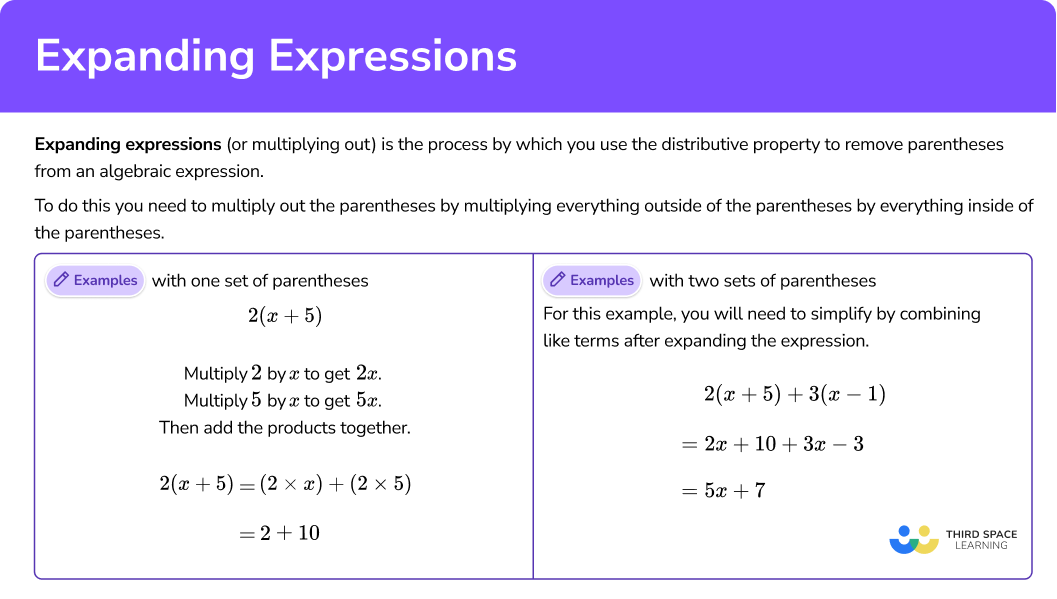# Expanding expressions

Here you will learn how to expand expressions before simplifying the resulting algebraic expressions.

Students will first learn about expanding expressions as part of expressions and equations in 6th grade.

## What is expanding expressions?

Expanding expressions (or multiplying out) is the process by which you use the distributive property to remove parentheses from an algebraic expression.

To do this, you need to multiply out the parentheses by multiplying everything outside of the parentheses by everything inside the parentheses. Then, if needed, you simplify the resulting expression by combining the like terms.

For example,

2(x+5) = 2x+10

For this example,

The expression has two sets of parentheses. You will need to simplify by combining like terms after expanding the expression.

\begin{aligned} & 2(x+5)+3(x-1) \\\\ & =2 x+10+3 x-3 \\\\ & =5 x+7 \end{aligned}

### What is expanding expressions?## Common Core State Standards

How does this relate to 6th grade math?

• Grade 6 – Expressions and Equations (6.EE.A.3)
Apply the properties of operations to generate equivalent expressions. For example, apply the distributive property to the expression 3 \, (2 + x) to produce the equivalent expression 6 + 3x; apply the distributive property to the expression 24x + 18y to produce the equivalent expression 6 \, (4x + 3y); apply properties of operations to y + y + y to produce the equivalent expression 3y .

## How to expand expressions

In order to expand expressions:

1. Use the distributive property to “multiply out” the parentheses in the expression.
2. Combine the like terms.

## Expanding expressions examples

### Example 1: one set of parentheses

Expand:

9 \, (y+6)

1. Use the distributive property to “multiply out” the parentheses in the expression.

\begin{aligned} & 9 \, (y+6) \\\\ & =(9 \times y)+(9 \times 6) \\\\ & =9 y+54 \end{aligned}

2Combine the like terms.

There are no like terms in the expression, so you are finished.

The expanded expression is 9y+54 .

### Example 2: one set of parentheses

Expand and simplify:

6 \, (𝑡 + 8)–12

Use the distributive property to “multiply out” the parentheses in the expression.

Combine the like terms.

### Example 3: one set of parentheses

5 b+7(b-2)-18

Use the distributive property to “multiply out” the parentheses in the expression.

Combine the like terms.

### Example 4: two sets of parentheses

Expand and simplify:

8(x-2)+6(x+3)

Use the distributive property to “multiply out” the parentheses in the expression.

Combine the like terms.

### Example 5: constants and variables outside of the parentheses

Expand and simplify:

2 x(x+6)-3(x-2)

Use the distributive property to “multiply out” the parentheses in the expression.

Combine the like terms.

### Example 6: variables in both terms in the parentheses

Expand and simplify:

3 \, (2 x-6 y)-5 \, (x-2 y)

Use the distributive property to “multiply out” the parentheses in the expression.

Combine the like terms.

### Teaching tips for expanding expressions

• Assess students’ prior knowledge before beginning. Review key vocabulary terms including algebraic expression, parentheses, simplify, exponents, distributive property, binomial, polynomial, terms, coefficients. Review adding and subtracting negative numbers as needed.

• Allowing learners to use templates or organizers when practicing this skill or completing worksheets, such as the area model shown above, will help them organize their work as they complete the process step-by-step.

• Be sure students have had plenty of practice expanding expressions with one set of parentheses before moving on to expanding expressions with binomials or polynomials.

• The expanding expression tool kit you will provide students is essential for higher levels of mathematics.

### Easy mistakes to make

• Multiplying all terms in the set of parentheses
You must multiply the term outside the parentheses by every term inside the parentheses.
For example,

2\left(6 x^2-3 x\right)=12 x^2-3 x

Here, you have not multiplied the value outside of the parentheses by the second term. The correct answer is:

2\left(6 x^2-3 x\right)=12 x^2-6 x

• Squaring a term
When you square something, you multiply it by itself.
For example,

\begin{aligned} 3^{2}&=3\times 3\\ x^{2}&=x\times x\\ (5y)^{2}&=5y\times 5y \end{aligned}

• Combining like terms
When you combine like terms, you must include the sign in front of the number. Be sure to underline the sign along with the number when combining the like terms.
For example,

### Practice expanding expressions questions

1. Expand and simplify:

5 \, (m+9)-4 m

5m+9m+99m+45m+45Expand each set of parentheses:

5m + 45-4m

Combine like terms:

m+45

2. Expand and simplify:

4 h-2(h+8)-1

2h-172h+172h-5h-15Expand each set of parentheses:

4h-2h-16-1

Combine like terms:

2h-17

3. Expand and simplify:

3 \, (x+7)-2 \, (x+3)

5x+15x+15x+275x+27Expand each set of parentheses:

3x + 21-2x-6

Combine like terms:

x+15

4. Expand and simplify:

8 \, (y-5)+5 \, (y-2)

13y-303y-5013y-503y-30Expand each set of parentheses:

8y-40 + 5y-10

Combine like terms:

13y-50

5. Expand and simplify:

5 x \, (3 x-2)-4 x \, (2 x+3)

23x^2-22x7x^2-57x^2-22x7x^2-2xExpand each set of parentheses:

15x^{2}-10x-8x^{2}-12x

Combine like terms:

7x^{2}-22x

6. Expand and simplify:

5 \, (6 x-2 y)-2 \, (8 x-5 y)

14x14x-20y46x14x+20yExpand each set of parentheses:

30x-10y-16x+10y

Combine like terms:

14x

## Expanding expressions FAQs

How do you expand an expression?

To expand an expression, you need to multiply out the parentheses by multiplying everything outside of the parentheses by everything inside of the parentheses. Then, if needed, you simplify the resulting expression by combining the like terms.

How do you combine like terms?

Combine like terms by first identifying the like terms, then adding them together using the value of their coefficients.

Why is expanding an expression an important skill to have?

Expanding expressions are useful when solving and graphing quadratic equations, rational expressions, and solving non-linear simultaneous equations.

## Still stuck?

At Third Space Learning, we specialize in helping teachers and school leaders to provide personalized math support for more of their students through high-quality, online one-on-one math tutoring delivered by subject experts.

Each week, our tutors support thousands of students who are at risk of not meeting their grade-level expectations, and help accelerate their progress and boost their confidence.